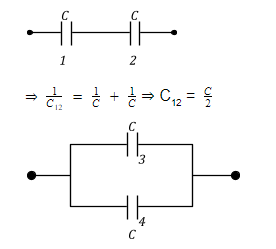# Two equal capacitors are first connected in series and then in parallel. The ratio of the equivalent capacities in the two cases will be

a. 2: 1

b. 1: 4

c. 4: 1

d. 1: 2

Given that first connectionSecond connection

C34 = C + C = 2 C

Now, the ratio of equivalent capacities in the two cases will be –

⇒ C12 / C34 = C2 / 2C ⇒ C12 / C34 = ¼(0)(0)